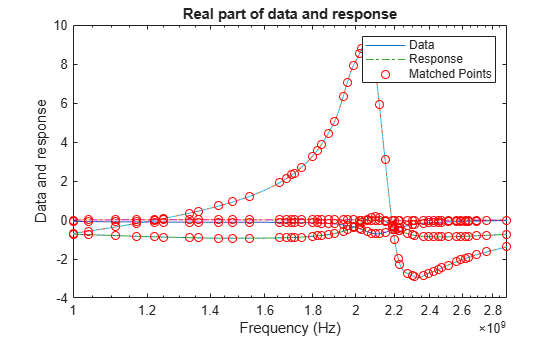# zpk

Compute zeros, poles, and gain of rational object

## Syntax

``[z,p,k,dcgain] = zpk(fit)``

## Description

example

````[z,p,k,dcgain] = zpk(fit)` returns the zeros, poles, gain, and DC gain of a rational object.```

## Examples

collapse all

Create an S-Parameters object from the file named `default.s2p`. Perform rational fitting of the S-Parameters.

```S = sparameters('default.s2p'); fit = rational(S,Display='plot')``````fit = rational with properties: NumPorts: 2 NumPoles: 44 Poles: [44x1 double] Residues: [2x2x44 double] DirectTerm: [2x2 double] ErrDB: -22.6464 ```

Calculate the zeros, poles, gain, and DC gain of the rational object.

`[z,p,k,dcgain] = zpk(fit)`
```z=2×2 cell array {43x1 double} {43x1 double} {43x1 double} {43x1 double} ```
```p=2×2 cell array {44x1 double} {44x1 double} {44x1 double} {44x1 double} ```
```k = 2×2 109 × -2.5743 -0.0556 -0.6980 -1.3221 ```
```dcgain = 2×2 0.0933 -0.0302 -1.1079 0.4746 ```

## Input Arguments

collapse all

Rational fit, specified as a `rational` object returned by `rational`.

## Output Arguments

collapse all

Zeroes of the fit, returned as a 1-D array of doubles or a 3-D array of doubles.

Poles of the fit, returned as a 1-D array of doubles or a 3-D array of doubles.

Gain of the fit, returned as a 2-D array of doubles. `k` is the coefficient of the rational function when poles and zeros are expressed as monic polynomials in `S`.

DC gain of the fit, returned as 2-D array of doubles for zero frequency response.

## Version History

Introduced in R2020a